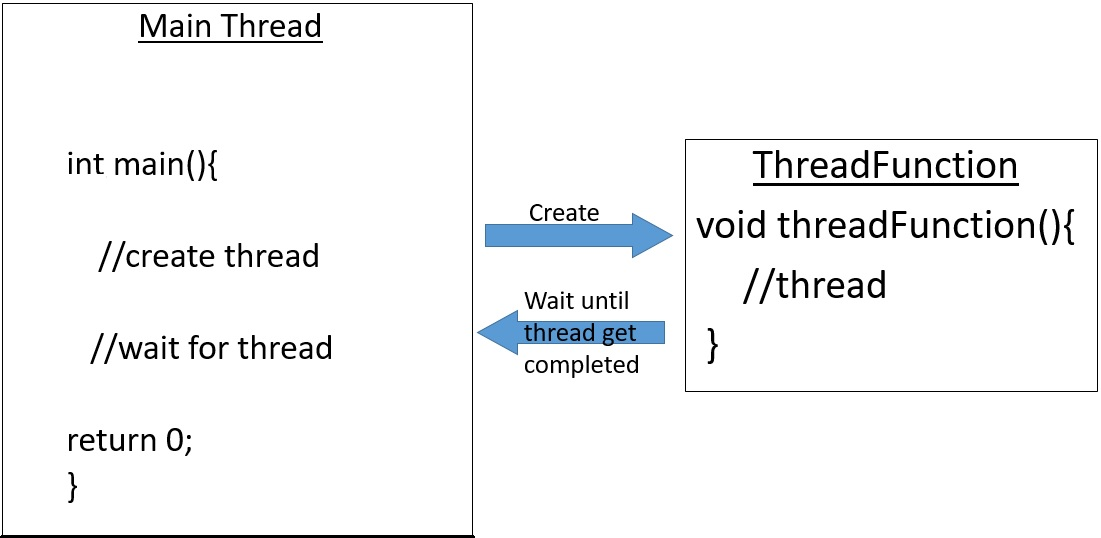×

Search anything:Reading time: 35 minutes | Coding time: 10 minutesIn main function we can create a thread which will have a entry point function the threadFunction() and we can wait for the thread to get completed and when the entry point function gets completed the control goes back to the main thread.

### Syntax

``````#include <thread>

int main(){
}
``````

We just need to include the thread library to create a thread. A simple example would be writing `thread t1` to create a thread named t1, additional options for the created thread can be passed as arguments to the constrctor already defined in the thread library.
Example -

``````thread t1 (threadFunction, valueToThreadFunction)
``````

• Process-based multitasking handles the concurrent execution of programs.
• Thread-based multitasking deals with the concurrent execution of pieces of the same program.

C++ does not contain any built-in support for multithreaded applications.Instead,it relies entirely upon the operating system to provide this feature.

When creating a thread,something need to be passed to be executed on it.A few things that can be passed to a thread:

• Free functions
• member functions
• Functor objects
• Lambda expressions

### Free function

Free function example - executes a function on a separate thread

``````#include <string>
#include <iostream>
using namespace std;

cout<<"value->"<<value<<endl;
}
int main(){

//wait for thread t1 to complete
t1.join();

return 0;
}
``````

### Member function

Member function example - executes a member function on a separate thread

``````#include <iostream>
using namespace std;

class fun
{
public:
{
cout <<"value->"<<value <<endl;
}
};

int main()
{
// Create and execute the thread

// The member function will be executed in a separate thread

// Wait for the thread to finish, this is a blocking operation
t1.join();
return 0;
}
``````

### Functor object

Functor object example

``````#include <iostream>
using namespace std;
class fun
{
public:
void operator()(int value)
{
cout<<"value->"<<value <<endl ;
}
};
int main()
{
// Create and execute the thread

// The functor object will be executed in a separate thread

// Wait for the thread to finish, this is a blocking operation
t1.join();
return 0;
}
``````

### Lambda expression

Lambda expression example

``````#include <iostream>
using namespace std;
int main()
{
auto lambda = [](int value) { cout<<"value->"<<value <<endl ; };
// Create and execute the thread

// The lambda expression will be executed in a separate thread

// Wait for thread to finish, this is a blocking operation
t1.join();
return  0;
}
``````

### Race condition

A race condition occurs when two or more threads can access shared data and they try to change it at the same time.In simple words, When two or more threads perform a set of operations in parallel, that access the same memory location. Also, one or more thread out of them modifies the data in that memory location, then this can lead to an unexpected results some times.Let's try to understand it with an example

``````class Wallet
{
int Money;
public:
Wallet() :Money(0){}
int getMoney() { return Money; }
for(int i = 0; i < money; ++i){
Money++;
}
}
};
``````

Now Let’s create 5 threads and all these threads will share a same object of class Wallet and add 1000 to internal money using it’s addMoney() member function in parallel.So if initially money in wallet is 0. Then after completion of all thread’s execution money in Wallet should be 5000.But as all threads are modifying the shared data at same time, it might be possible that in some scenarios money in wallet at end will be much lesser than 5000.

``````int testMultithreadedWallet(){
Wallet walletObject;
for(int i = 0; i < 5; ++i){
}
for(int i = 0; i < threads.size() ; i++){
}
return walletObject.getMoney();
}

int main(){
int val = 0;
for(int k = 0; k < 1000; k++){
std::cout << "Error at count = "<<k<<" Money in Wallet = "<<val << std::endl;
}
}
return 0;
}
``````

As addMoney() member function of same Wallet class object is executed 5 times hence it’s internal money is expected to be 5000. But as addMoney() member function is executed in parallel hence in some scenarios Money will be much lesser than 5000 i.e. output is

``````Error at count = 971  Money in Wallet = 4568                                         Error at count = 971  Money in Wallet = 4568                                         Error at count = 972  Money in Wallet = 4260                                    Error at count = 972  Money in Wallet = 4260                                    Error at count = 973  Money in Wallet = 4976                                          Error at count = 973  Money in Wallet = 4976
``````

This is a race condition, as here two or more threads were trying to modify the same memory location at same time and lead to unexpected result.

### How to fix Race Conditions?

To fix this problem we need to use Lock mechanism i.e. each thread need to acquire a lock before modifying or reading the shared data and after modifying the data each thread should unlock the Lock.

### Mutex

The mutex class is a synchronization primitive that can be used to protect shared data from being simultaneously accessed by multiple threads.C++ offers a selection of mutex classes:

• std::mutex - offers simple locking functionality.
• std::timed_mutex - offers try_to_lock functionality
• std::recursive_mutex - allows recursive locking by the same thread.
• std::shared_mutex, std::shared_timed_mutex - offers shared and unique lock
functionalities
``````#include <iostream>
#include <mutex>
#include <shared_mutex>
#include <chrono>

using namespace std;

lock_guard<mutex> lock(mtx);
cout<<"Mutex is locked"<<endl;
}

int main(){
mutex mtx;
unique_lock<mutex> lock(mtx);